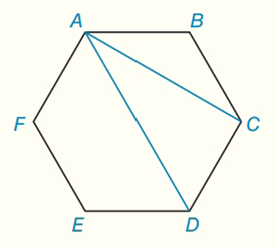Chapter 7.CT, Problem 15CTElementary Geometry For College St...

7th Edition
Alexander + 2 others
ISBN: 9781337614085

Solutions

Chapter
SectionElementary Geometry For College St...

7th Edition
Alexander + 2 others
ISBN: 9781337614085
Textbook Problem

For a regular hexagon ABCDEF, the length of side A B ¯ is 4 in. Find the exact length ofa) diagonal A C . ¯ b) diagonal A D . ¯To determine

(a)

To find:

The exact length of diagonal AC¯ for a regular hexagon.

Explanation

Given:

For a regular hexagon ABCDEF, the length of side AB¯ is 4 in.

Calculation:

To find: the exact length of diagonal AC¯ for a regular hexagon.

We connect point B to the midpoint, M, of AC it splits ABC into two 30 60 90 triangles.

To determine

(b)

To find:

The exact length of diagonal AD¯ for a regular hexagon.

Still sussing out bartleby?

Check out a sample textbook solution.

See a sample solution

The Solution to Your Study Problems

Bartleby provides explanations to thousands of textbook problems written by our experts, many with advanced degrees!

Get Started

Solve for x, assuming a, b, and c are negative constants. 60. ax+bcb

Single Variable Calculus: Early Transcendentals, Volume I

g(x)=2(x1)5/2

Applied Calculus for the Managerial, Life, and Social Sciences: A Brief Approach

Fill in each blank: 72ft=yd

Elementary Technical Mathematics

If f(2) = 10 and f'(x) = x2f(x) for all x, find f"(2).

Single Variable Calculus: Early Transcendentals

True or False: converges.

Study Guide for Stewart's Multivariable Calculus, 8th

For y = sin 6x, dydx=. a) cos 6x b) 6 cos x c) cos 6 d) 6 cos 6x

Study Guide for Stewart's Single Variable Calculus: Early Transcendentals, 8th